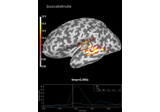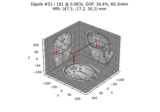# mne.inverse_sparse.mixed_norm¶

mne.inverse_sparse.mixed_norm(evoked, forward, noise_cov, alpha, loose='auto', depth=0.8, maxit=3000, tol=0.0001, active_set_size=10, debias=True, time_pca=True, weights=None, weights_min=0.0, solver='auto', n_mxne_iter=1, return_residual=False, return_as_dipoles=False, dgap_freq=10, rank=None, pick_ori=None, verbose=None)[source]

Mixed-norm estimate (MxNE) and iterative reweighted MxNE (irMxNE).

Compute L1/L2 mixed-norm solution 1 or L0.5/L2 2 mixed-norm solution on evoked data.

Parameters
evokedinstance of `Evoked` or `list` of instances of `Evoked`

Evoked data to invert.

forward`dict`

Forward operator.

noise_covinstance of `Covariance`

Noise covariance to compute whitener.

alpha`float` in `range` [0, 100)

Regularization parameter. 0 means no regularization, 100 would give 0 active dipole.

loose`float` | ‘auto’ | `dict`

Value that weights the source variances of the dipole components that are parallel (tangential) to the cortical surface. Can be:

• float between 0 and 1 (inclusive)

If 0, then the solution is computed with fixed orientation. If 1, it corresponds to free orientations.

• `'auto'` (default)

Uses 0.2 for surface source spaces (unless `fixed` is True) and 1.0 for other source spaces (volume or mixed).

• dict

Mapping from the key for a given source space type (surface, volume, discrete) to the loose value. Useful mostly for mixed source spaces.

depth

How to weight (or normalize) the forward using a depth prior. If float (default 0.8), it acts as the depth weighting exponent (`exp`) to use None is equivalent to 0, meaning no depth weighting is performed. It can also be a `dict` containing keyword arguments to pass to `mne.forward.compute_depth_prior()` (see docstring for details and defaults). This is effectively ignored when `method='eLORETA'`.

Changed in version 0.20: Depth bias ignored for `method='eLORETA'`.

maxit`int`

Maximum number of iterations.

tol`float`

Tolerance parameter.

active_set_size

Size of active set increment. If None, no active set strategy is used.

debiasbool

Remove coefficient amplitude bias due to L1 penalty.

time_pca

If True the rank of the concatenated epochs is reduced to its true dimension. If is ‘int’ the rank is limited to this value.

weights

Weight for penalty in mixed_norm. Can be None, a 1d array with shape (n_sources,), or a SourceEstimate (e.g. obtained with wMNE, dSPM, or fMRI).

weights_min`float`

Do not consider in the estimation sources for which weights is less than weights_min.

solver‘prox’ | ‘cd’ | ‘bcd’ | ‘auto’

The algorithm to use for the optimization. ‘prox’ stands for proximal iterations using the FISTA algorithm, ‘cd’ uses coordinate descent, and ‘bcd’ applies block coordinate descent. ‘cd’ is only available for fixed orientation.

n_mxne_iter`int`

The number of MxNE iterations. If > 1, iterative reweighting is applied.

return_residualbool

If True, the residual is returned as an Evoked instance.

return_as_dipolesbool

If True, the sources are returned as a list of Dipole instances.

dgap_freq

The duality gap is evaluated every dgap_freq iterations. Ignored if solver is ‘cd’.

rank`None` | ‘info’ | ‘full’ | `dict`

This controls the rank computation that can be read from the measurement info or estimated from the data. When a noise covariance is used for whitening, this should reflect the rank of that covariance, otherwise amplification of noise components can occur in whitening (e.g., often during source localization).

`None`

The rank will be estimated from the data after proper scaling of different channel types.

`'info'`

The rank is inferred from `info`. If data have been processed with Maxwell filtering, the Maxwell filtering header is used. Otherwise, the channel counts themselves are used. In both cases, the number of projectors is subtracted from the (effective) number of channels in the data. For example, if Maxwell filtering reduces the rank to 68, with two projectors the returned value will be 66.

`'full'`

The rank is assumed to be full, i.e. equal to the number of good channels. If a `Covariance` is passed, this can make sense if it has been (possibly improperly) regularized without taking into account the true data rank.

`dict`

Calculate the rank only for a subset of channel types, and explicitly specify the rank for the remaining channel types. This can be extremely useful if you already know the rank of (part of) your data, for instance in case you have calculated it earlier.

This parameter must be a dictionary whose keys correspond to channel types in the data (e.g. `'meg'`, `'mag'`, `'grad'`, `'eeg'`), and whose values are integers representing the respective ranks. For example, `{'mag': 90, 'eeg': 45}` will assume a rank of `90` and `45` for magnetometer data and EEG data, respectively.

The ranks for all channel types present in the data, but not specified in the dictionary will be estimated empirically. That is, if you passed a dataset containing magnetometer, gradiometer, and EEG data together with the dictionary from the previous example, only the gradiometer rank would be determined, while the specified magnetometer and EEG ranks would be taken for granted.

The default is `None`.

New in version 0.18.

pick_ori`None` | “normal” | “vector”

Options:

• `None`

Pooling is performed by taking the norm of loose/free orientations. In case of a fixed source space no norm is computed leading to signed source activity.

• `"normal"`

Only the normal to the cortical surface is kept. This is only implemented when working with loose orientations.

• `"vector"`

No pooling of the orientations is done, and the vector result will be returned in the form of a `mne.VectorSourceEstimate` object.

verbose

If not None, override default verbose level (see `mne.verbose()` and Logging documentation for more). If used, it should be passed as a keyword-argument only.

Returns
stc

Source time courses for each evoked data passed as input.

residualinstance of `Evoked`

The residual a.k.a. data not explained by the sources. Only returned if return_residual is True.

References

1

Alexandre Gramfort, Matthieu Kowalski, and Matti S. Hämäläinen. Mixed-norm estimates for the M/EEG inverse problem using accelerated gradient methods. Physics in Medicine and Biology, 57(7):1937–1961, 2012. doi:10.1088/0031-9155/57/7/1937.

2

Daniel Strohmeier, Yousra Bekhti, Jens Haueisen, and Alexandre Gramfort. The iterative reweighted mixed-norm estimate for spatio-temporal MEG/EEG source reconstruction. IEEE Transactions on Medical Imaging, 35(10):2218–2228, 2016. doi:10.1109/TMI.2016.2553445.

## Examples using `mne.inverse_sparse.mixed_norm`¶